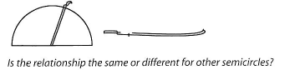Lesson 14 Tents

# Episode 2

Reasoning Resources: Worksheet 2 (optional), large paper circle (optional), compasses (optional), rulers or measuring tapes, prepared semicircles (optional), calculators
Whole class preparation
Moving to quantifying the relationship in ratio terms but without measurement and calculation.

Using uncalibrated string, flexible strips, etc. avoids work with numbers and focuses the challenge to a comparison of unmeasured lengths where one is approximately a whole number of times the other.Having established that the radius is the key feature of a semicircle ask: How many times the radius is the curve of the semicircle? Show, on a large drawing on the board, a possible way of marking a piece of string with the height (radius) of the semicircle. How can you compare this to the length of the curve of the semicircle? Record ideas on the board. Demonstrating a single method on the board ask: How many times greater is the length of the semicircle curve than the radius? Possible answers:‘around 3 times’ or ‘3 and a half times’. Is the relationship the same or different for other semicircles?
Pair and small group work I
Pupils find their ratio directly by comparing unmeasured lengths, then through measurements, grounding the concept in their mental frameworks through repeated confirmation.

Give out lengths of string, flexible strips (card or plastic or metal strips which are springy, or curtain wire) and ask pupils to use these or other ideas to explore and justify the link between the radius and the length of the curve of the semicircle.

Pupils can sketch or draw the semicircles using compasses, or use prepared semicircles made from card, plywood or plastic. You may wish to give pupils Worksheet 2, to help them structure their work.

Teacher mediation in this phase will challenge premature conclusions, e.g. that the semicircle is twice the length of the radius, or pupils’ ways of explaining their thinking.
Whole class sharing and discussion
Another experience of ‘transition from additive thinking to the relational thinking of proportionality.

When groups begin to see the idea of a possibly constant ratio for radius to arc length, the teacher can suggest calibrated measurements to establish the ratio numerically.
Collect ideas on the relationship between the radius and semicircle arc in pupils’ words, establishing that it seems roughly the same for different sized semicircles. Clarify the ideas of ‘roughly three times the radius’ and why an error of ‘twice’ can emerge. It is possible pupils may mean ‘twice the radius more, which means ‘three times’ but explained as addition.

The 'three and a bit’ value of the ratio of semicircle arc length to radius could emerge through variations of ’3 and a half’’3 and a third’. Some pupils may recall the learned value of Pi, and that should be aired without being made into the aim of the activity.
Pair and small group work II

Measurements remain approximate with whatever accuracy, but calculations, especially with calculators, are exact.

The difference between exactness and high degree of accuracy for irrational numbers is accessible to some pupils.
Steer pupils’ attention now towards accuracy. The differing values for the ‘three and a bit’ arise from the variation in accuracy of measurement, and more accurate values come from larger circles. You may wish to demonstrate this with a large paper circle, showing by folding that the ‘bit’ extra is less than a quarter, but perhaps more than one eighth of the radius.

Give out rulers, or tapes for pupils to measure the lengths of string/strips for their semicircles and calculate, using numbers and calculators, the ratio length of semicircle curve radius.
Sharing values for Pi
Collect the different values to any degree of accuracy. Then switch attention to the actual value of the decimal part as a fraction, and the convenience of 3 1/7 or 22/7 and how to understand it as slightly more than ‘3, and why an 1/8 is convenient for understanding (half of 1/4), while the decimal notation is more complicated. Some pupils would realise the need for accuracy to a large number of decimal places in buildings, scientific and astronomical work. Irrational vs. rational number discussion is possible in some cases.